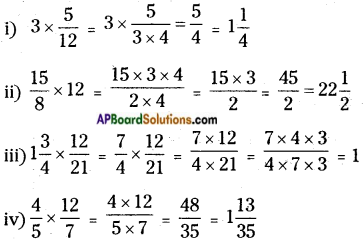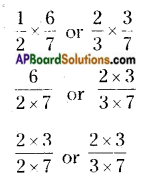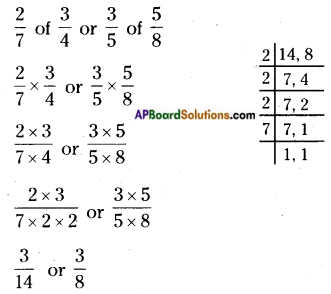# AP Board 6th Class Maths Solutions Chapter 5 Fractions and Decimals Ex 5.2

AP State Syllabus AP Board 6th Class Maths Solutions Chapter 5 Fractions and Decimals Ex 5.2 Textbook Questions and Answers.

## AP State Syllabus 6th Class Maths Solutions 5th Lesson Fractions and Decimals Ex 5.2

Question 1.
Find the product of the following.
i) 3 × $$\frac{5}{12}$$
ii) $$\frac{15}{8}$$ × 12
iii) 1$$\frac{3}{4}$$ × $$\frac{12}{21}$$
iv) $$\frac{4}{5}$$ × $$\frac{12}{7}$$Question 2.
Which is greater?
i) $$\frac{1}{2}$$ of $$\frac{6}{7}$$ or $$\frac{2}{3}$$ of $$\frac{3}{7}$$
ii) $$\frac{2}{7}$$ of $$\frac{3}{4}$$ or $$\frac{3}{5}$$ of $$\frac{5}{8}$$
i) $$\frac{1}{2}$$ of $$\frac{6}{7}$$ or $$\frac{2}{3}$$ of $$\frac{3}{7}$$$$\frac{3}{7}$$ or $$\frac{2}{7}$$
So, $$\frac{3}{7}$$ > $$\frac{2}{7}$$
∴ $$\frac{1}{2}$$ of $$\frac{6}{7}$$ > $$\frac{2}{3}$$ of $$\frac{3}{7}$$

ii) $$\frac{2}{7}$$ of $$\frac{3}{4}$$ or $$\frac{3}{5}$$ of $$\frac{5}{8}$$Convert them into like fractions
LCM of denominators = 2 × 2 × 2 × 7 = 56
$$\frac{3 \times 4}{14 \times 4}$$ or $$\frac{3 \times 7}{8 \times 7}$$
$$\frac{12}{56}$$ or $$\frac{21}{56}$$
So, $$\frac{12}{56}$$ < $$\frac{21}{56}$$
∴ $$\frac{2}{7}$$ of $$\frac{3}{4}$$ < $$\frac{3}{5}$$ of $$\frac{5}{8}$$Question 3.
Find:
i) $$\frac{7}{11}$$ of 330
ii) $$\frac{5}{9}$$ of 108
iii) $$\frac{2}{7}$$ of 16
iv) $$\frac{1}{7}$$ of $$\frac{3}{10}$$
i) $$\frac{7}{11}$$ of 330
= $$\frac{7}{11}$$ × 330
= $$\frac{7}{11}$$ × 11 × 30
= 7 × 30 = 210

ii) $$\frac{5}{9}$$ of 108
= $$\frac{5}{9}$$ × 108
= $$\frac{5}{9}$$ × 9 × 12
= 5 × 12 = 60

iii) $$\frac{2}{7}$$ of 16
= $$\frac{2}{7}$$ × 16
= $$\frac{2×16}{7}$$
= $$\frac{32}{7}$$ or 4$$\frac{4}{7}$$

iv) $$\frac{1}{7}$$ of $$\frac{3}{10}$$
= $$\frac{1}{7}$$ × $$\frac{3}{10}$$
= $$\frac{1 \times 3}{7 \times 10}$$
= $$\frac{3}{70}$$

Question 4.
If the cost of a notebook is Rs. 10$$\frac{3}{4}$$. Then, find the cost of 36 books.
Cost of each notebook = Rs. 10$$\frac{3}{4}$$ (or) $$\frac{43}{4}$$
Cost of 36 notebooks = 36 × 10$$\frac{3}{4}$$ = 36 × $$\frac{43}{4}$$ = $$\frac{9 \times 4 \times 43}{4}$$ = 9 × 43
Cost of 36 notebooks = Rs. 387Question 5.
A motor bike runs 52$$\frac{1}{2}$$ km using 1 litre of petrol. How much distance will it cover for 2$$\frac{3}{4}$$ litres of petrol ?
Distance covered by the motor bike 1 litre petrol = 52$$\frac{1}{2}$$ km (or) $$\frac{105}{2}$$ km
Distance covered by the motor bike for 2$$\frac{3}{4}$$ litres of petrol = 2$$\frac{3}{4}$$ of 52$$\frac{1}{2}$$
= $$\frac{11}{4}$$ × $$\frac{105}{2}$$
= $$\frac{11 \times 105}{4 \times 2}$$
∴ Distance covered by the motor bike for 2$$\frac{3}{4}$$ litres of petrol = $$\frac{1155}{8}$$ = 144$$\frac{3}{8}$$ km.